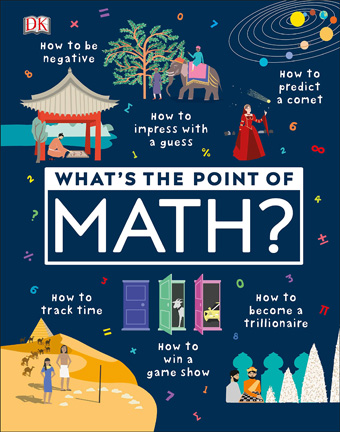# What's the Point of Math

## by DK

\$25.00

Format: Tactile (Braille)

Item: POINT-OF-MATH
In 3 volumes
In UEB; includes 93 tactile illustrations
Ages 9 — 12 years

Math makes the world go around!

What's The Point of Math? unpacks how math is an essential part of our everyday life — in many surprising ways! It helps bring numbers and mathematical explanations to life with crazy facts, magic tricks, mathematical brainteasers, and tactile illustrations, and the "Try it out" examples give mathematical explanations that are simple and easy to grasp. Kids will learn about how the formation of number sequences began, the origins of trigonometry, and how to become a trillionaire!

What's the Point of Math? explains:

• Numbers and counting
• Shapes and measuring
• Patterns and sequences
• Probability and logic
• Data and statistics

What's the Point of Math? will change kids' perception of numbers and give them the skills and understanding to apply the principles in their everyday life. Show them that math is interesting, fun — and not intimidating at all!